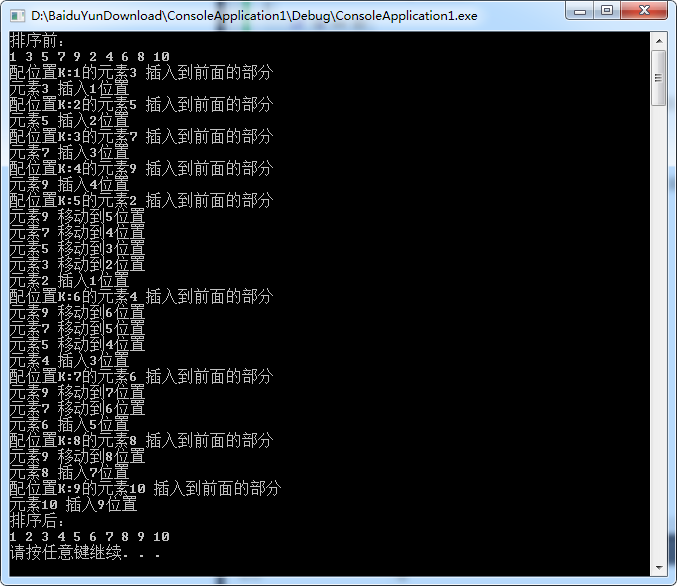2020-03-12 11:35

# 数组排序函数，看不太懂 求大神解答

void inserSort(int *a,int k)
{
if(k==0)
return;
//对 K-1 个元素排序；
inserSort(a,k-1);
//配位置K的元素 插入到前面的部分;
int x=a[k];
int index =k-1;
while(index>-1&&x<a[index])
{
a[index+1]=a[index];
index--;
}
a[index+1]=x;
}

• 点赞
• 写回答
• 关注问题
• 收藏
• 邀请回答

#### 3条回答默认 最新

•白色一大坨 2020-03-12 12:25
已采纳

这是个递归程序，每次找到一个数，会把它和前面的数进行比较，有比它大的就进行交换，不断运行直到排序完毕，我给你加了些log，你可以自己运行一下看看：

``````#include <stdio.h>
#include <stdlib.h>
#define N 10
void inserSort(int *a, int k)
{
if (k == 0)
return;
//对 K-1 个元素排序；
inserSort(a, k - 1);
//配位置K的元素 插入到前面的部分;
printf("配位置K:%d的元素%d 插入到前面的部分\n",k, a[k]);
int x = a[k];
int index = k - 1;
while (index > -1 && x < a[index])
{
printf("元素%d 移动到%d位置\n", a[index], index + 1);
a[index + 1] = a[index];
index--;
}

printf("元素%d 插入%d位置\n", x, index + 1);
a[index + 1] = x;
}

void main()
{
int i;
int a[N] = { 1, 3, 5, 7, 9, 2,4,6,8,10 };
printf("排序前：\n");
for (i = 0; i < N; i++)
{
printf("%d ", a[i]);
}
printf("\n");
inserSort(a, N-1);
printf("排序后：\n");
for (i = 0; i < N;i++)
{
printf("%d ", a[i]);
}

printf("\n");
system("pause");
}
``````点赞 评论
•从小到大排序的

``````int arr = {8, 9, 5, 6, 4, 7, 2, 3, 1, 0};
inserSort(arr, 10);
for (int i=0; i<10; i++) {

qDebug() << "i:::" << i << arr[i];
}
``````

i::: 0 0
i::: 1 1
i::: 2 2
i::: 3 3
i::: 4 4
i::: 5 5
i::: 6 6
i::: 7 7
i::: 8 8
i::: 9 9

点赞 评论
•blownewbee 2020-03-12 12:42

这就是插入排序，就是故意把循环写成递归而已

``````void inserSort(int *a,int k)
{
while (1)
{
if (k == 0)
break; // return; 或者你可以把这行和上面一行合并，写while (k > 0)
//对 K-1 个元素排序；
k = k - 1; //inserSort(a, k - 1)
//配位置K的元素 插入到前面的部分;
int x=a[k];
int index =k-1;
while(index>-1&&x<a[index])
{
a[index+1]=a[index];
index--;
}
a[index+1]=x;
}
}
``````

这是通常的写法。

再体会下，比如我有一个冒泡排序

``````void buSort(int *a,int k)
{
for (int i = 0; i < k - 1; i++)
{
for (int j = 0; j < k - i - 1; j++)
{
if (a[j] > a[j + 1])
{
int temp = a[j];
a[j] = a[j + 1];
a[j + 1] = temp;
}
}
}
}
``````

我也可以把循环藏起来：

``````void buSort(int *a,int k, int i = 0, int j = 0)
{
if (i >= k - 1) return;
if (a[j] > a[j + 1])
{
int temp = a[j];
a[j] = a[j + 1];
a[j + 1] = temp;
}
i++;
j++;
if (j >=  k - i - 1) j = 0;
buSort(a, k, i, j);
}
``````

如果你觉得困难，再看一个简单的

``````int sum(int n)
{
int s = 0, i = 1;
while (i <= 100) s += i++;
return s;
}
``````

这是一个计算1+2+...+n的代码
它也可以把while改写

``````int sum(int n, int s = 0, int i = 1)
{
if (i > 100) return s;
s += i++;
return sum(n, s, i);
}
``````

总之，任何循环都可以改写为递归，你只要掌握规律就能看懂这种故弄玄虚的代码。

点赞 评论